# Extension of a group

(diff) ← Older revision | Latest revision (diff) | Newer revision → (diff)

A group containing the given group as a normal subgroup. The quotient group is usually prescribed as well, that is, an extension of a groupby a groupis a groupcontainingas a normal subgroup and such that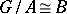, i.e. it is an exact sequence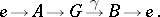(1)

In the literature other terminology is sometimes adopted, e.g.,may be called an extension ofby(see , for example), the epimorphism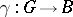itself may be called an extension of(see ), or the exact sequence (1) may be called an extension ofby, or an extension ofby. An extension ofbyalways exists, although it is not uniquely determined byand. The need to describe all extensions ofbyup to a natural equivalence is motivated by the demands both of group theory itself and of its applications. Two extensions ofbyare called equivalent if there is a commutative diagram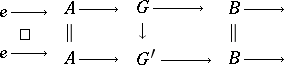Any extension of the form (1) determines, via conjugation of the elements of the group, a homomorphism, where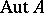is the automorphism group of,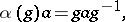such that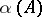is contained in the group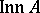of inner automorphisms of. Henceinduces a homomorphismThe triple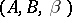is called the abstract kernel of the extension. Given an extension (1), one chooses for every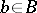a representativein such a way that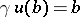and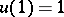. Then conjugation bydetermines an automorphism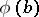of,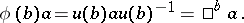The product of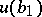and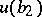is equal to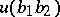up to a factor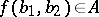:It is easily checked that these functions must satisfy the conditions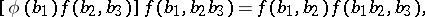(2)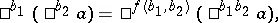(3)

where the function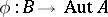is implicit in (3).

Given groupsandand functions,satisfying (2), (3) and the normalization conditionsone can define an extension (1) in the following way. The product set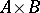is a group under the operationThe homomorphisms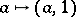,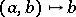yield an extension.

Given an abstract kernel, it is always possible to find a normalized functionsatisfying condition (3). A functionarises naturally, but condition (2) is not always fulfilled. In general,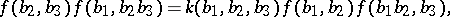where. The function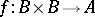is called a factor set and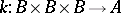is called the obstruction to the extension. If the groupis Abelian, then the factor sets form a group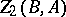under natural composition. Factor sets corresponding to a semi-direct product form a subgroupof. The quotient group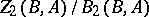is isomorphic to the second cohomology group ofwith coefficients in. Obstructions have a similar interpretation in the third cohomology group.

The idea of studying extensions by means of factor sets appeared long ago (O. Hölder, 1893). However, the introduction of factor sets is usually connected with the name of O. Schreier, who used them to undertake the first systematic study of extensions. R. Baer was the first to carry out an invariant study of group extensions without using factor sets. The theory of group extensions is one of the cornerstones of homological algebra.

How to Cite This Entry:
Extension of a group. Encyclopedia of Mathematics. URL: http://encyclopediaofmath.org/index.php?title=Extension_of_a_group&oldid=13641
This article was adapted from an original article by V.E. Govorov (originator), which appeared in Encyclopedia of Mathematics - ISBN 1402006098. See original article